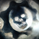1331 görüntülenme
1331
This Indicator is yet another variation of KC. Inspired from Value Charts webinar. I have seen their videos on youtube. What appears to be a variation of KC.
They use 12 bands Showing the zone, and different MA for different timeframes.

You can get this indicator close to accuracy by changing the inputs (ATR) and (Deviations)

This also can be used with the triple ATR setup - Change the values to EMA to desired value. To obtain the First band to plot @ 1, Change the Band deviation to 0.25.

Also can be used as as the Acceleration Band. With Current Settings, the Third Band will plot the Acceleration band.

List of All my Indicators - https://www.tradingview.com/p/stocks/?so...
Lycka Till
// Created by UCSgears
// This is a variation to what value charts uses.
// I have not seen their indicator code, But This is my interpretation, After all its just an indicator.

study(title="UCS_Value Band", shorttitle="VB_UCS", overlay=true)
source = close
length = input(21, minval=1, title = "EMA Length")
atrlen = input(5, minval=1, title = "ATR Length")
atrlen2 = round(atrlen*1.5)
atrlen3 = atrlen*2

mult = input(0.2, minval=0.1, title = "Band Deviation")
ma = sma(source, length)
range =  tr
rangema1 = ema(range, atrlen)
rangema2 = ema(range, atrlen2)
rangema3 = ema(range, atrlen3)

up1 = ma + rangema1 * mult*1
up2 = ma + rangema1 * mult*2
up3 = ma + rangema1 * mult*3
up4 = ma + rangema1 * mult*4
up5 = ma + rangema2 * mult*5
up6 = ma + rangema2 * mult*6
up7 = ma + rangema2 * mult*7
up8 = ma + rangema2 * mult*8
up9 = ma + rangema3 * mult*9
up10 = ma + rangema3 * mult*10
up11 = ma + rangema3* mult*11
up12 = ma + rangema3 * mult*12

dn1 = ma - rangema1 * mult*1
dn2 = ma - rangema1 * mult*2
dn3 = ma - rangema1 * mult*3
dn4 = ma - rangema1 * mult*4
dn5 = ma - rangema2 * mult*5
dn6 = ma - rangema2 * mult*6
dn7 = ma - rangema2 * mult*7
dn8 = ma - rangema2 * mult*8
dn9 = ma - rangema3 * mult*9
dn10 = ma - rangema3 * mult*10
dn11 = ma - rangema3* mult*11
dn12 = ma - rangema3 * mult*12

plot(ma, color=aqua, title="Basis")

color1 = green
color2 = yellow
color3 = red

//plot(up1, color = color1)
//plot(up2, color = color1)
//plot(up3, color = color1)
u4 = plot(up4, color = color1, title="Fair Value Upper")
//plot(up5, color = color2)
//plot(up6, color = color2)
//plot(up7, color = color2)
u8 = plot(up8, color = color2, title="High Value Upper")
//plot(up9, color = color3)
//plot(up10, color = color3)
//plot(up11, color = color3)
u12 = plot(up12, color = color3, title="Extreme Value Upper")

//plot(dn1, color = color1)
//plot(dn2, color = color1)
//plot(dn3, color = color1)
d4 = plot(dn4, color = color1, title="Fair Value Lower")
//plot(dn5, color = color2)
//plot(dn6, color = color2)
//plot(dn7, color = color2)
d8 = plot(dn8, color = color2, title="High Value Lower")
//plot(dn9, color = color3)
//plot(dn10, color = color3)
//plot(dn11, color = color3)
d12 = plot(dn12, color = color3, title="Extreme Value Lower")

fill (u4,d4,green)
fill (u4,u8, yellow)
fill (d4,d8, yellow)
fill (u8,u12, red)
fill (d8,d12, red)http://chartandplay.blogspot.com/2014/10/sometimes-free-indicators-with-price.html - Why Pay when you can find it free?

This is directly comparable to Value Charts - Value Bands. follow the link for proof
Cevap GönderAfter Exploring the possibility of using this Value Bands. When does this make real sense to use this? Ofcourse not while the Momentum is large.

IMHO, i do think it better used in a Squeeze, While keeping the Squeeze (20,2,1.5) does not give you enough edge to profit. So based on a little experimentation I think it is best applied in a Wider Squeeze. Answer to how wide the squeeze must be is here.

I am planning to improve this indicator by cleaning the bands up. Until then, UCSgears
Cevap GönderUse this setting for Daily Chart on Options (5, 5, 0.2)
Cevap Gönder